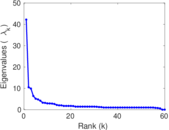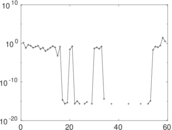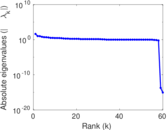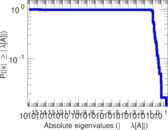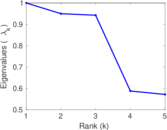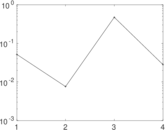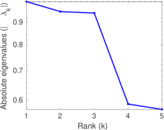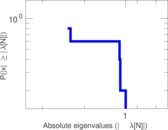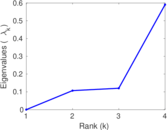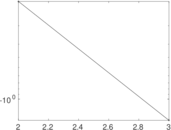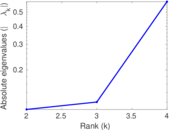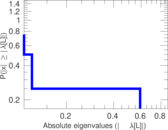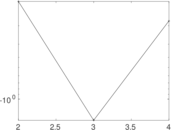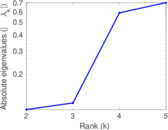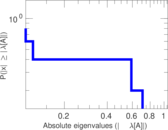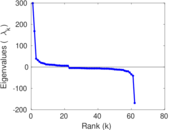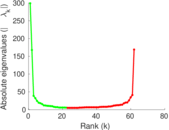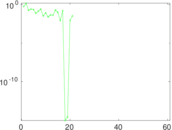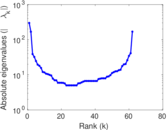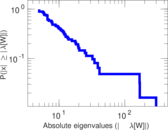# Wikibooks edits (tk)

This is the bipartite edit network of the Turkmen Wikibooks. It contains users and pages from the Turkmen Wikibooks, connected by edit events. Each edge represents an edit. The dataset includes the timestamp of each edit.

 Code `btk` Internal name `edit-tkwikibooks` Name Wikibooks edits (tk) Data source http://dumps.wikimedia.org/ AvailabilityDataset is available for download Consistency checkDataset passed all tests Category Authorship network Dataset timestamp 2017-10-20 Node meaning User, article Edge meaning Edit Network formatBipartite, undirected Edge typeUnweighted, multiple edges Temporal dataEdges are annotated with timestamps

## Statistics

 Size n = 307 Left size n1 = 62 Right size n2 = 245 Volume m = 373 Unique edge count m̿ = 283 Wedge count s = 5,222 Claw count z = 141,637 Cross count x = 3,195,945 Square count q = 176 4-Tour count T4 = 22,898 Maximum degree dmax = 100 Maximum left degree d1max = 100 Maximum right degree d2max = 42 Average degree d = 2.429 97 Average left degree d1 = 6.016 13 Average right degree d2 = 1.522 45 Fill p = 0.018 630 7 Average edge multiplicity m̃ = 1.318 02 Size of LCC N = 109 Diameter δ = 7 50-Percentile effective diameter δ0.5 = 1.571 92 90-Percentile effective diameter δ0.9 = 3.194 25 Median distance δM = 2 Mean distance δm = 2.240 71 Gini coefficient G = 0.583 491 Balanced inequality ratio P = 0.269 437 Left balanced inequality ratio P1 = 0.209 115 Right balanced inequality ratio P2 = 0.388 740 Relative edge distribution entropy Her = 0.858 779 Power law exponent γ = 5.196 24 Tail power law exponent γt = 2.661 00 Tail power law exponent with p γ3 = 2.661 00 p-value p = 0.006 000 00 Left tail power law exponent with p γ3,1 = 2.061 00 Left p-value p1 = 0.642 000 Right tail power law exponent with p γ3,2 = 3.421 00 Right p-value p2 = 0.000 00 Degree assortativity ρ = −0.221 759 Degree assortativity p-value pρ = 0.000 169 247 Spectral norm α = 42.201 9 Algebraic connectivity a = 0.107 002 Spectral separation |λ1[A] / λ2[A]| = 4.022 23 Controllability C = 186 Relative controllability Cr = 0.615 894

## Plots

### Fruchterman–Reingold graph drawing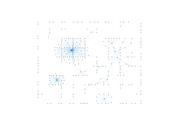### Degree distribution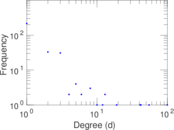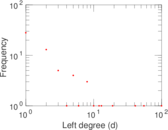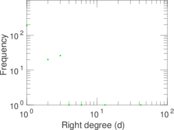### Cumulative degree distribution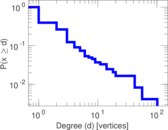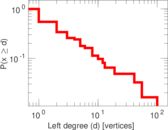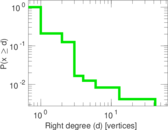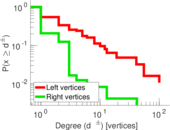### Lorenz curve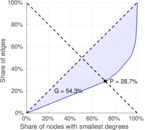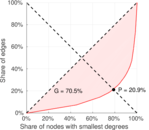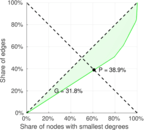### Spectral distribution of the adjacency matrix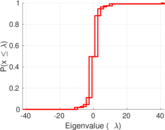### Spectral distribution of the normalized adjacency matrix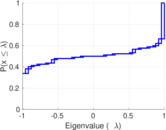### Spectral distribution of the Laplacian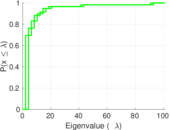### Spectral graph drawing based on the adjacency matrix### Spectral graph drawing based on the Laplacian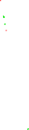### Spectral graph drawing based on the normalized adjacency matrix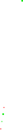### Degree assortativity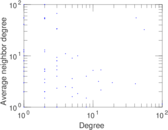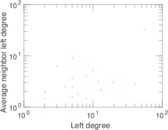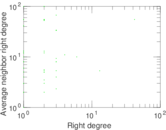### Zipf plot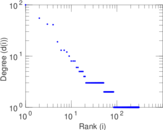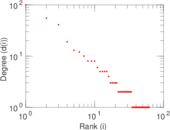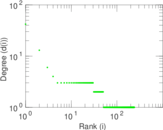### Hop distribution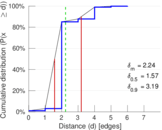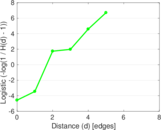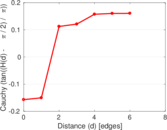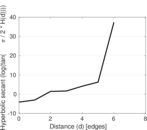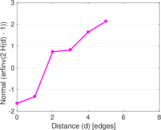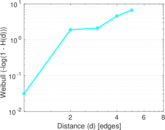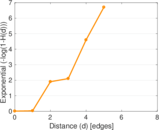### Double Laplacian graph drawing### Delaunay graph drawing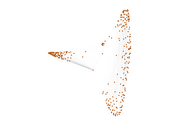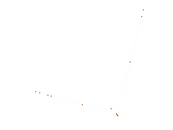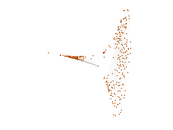### Edge weight/multiplicity distribution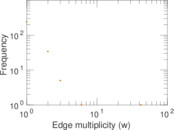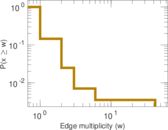### Temporal distribution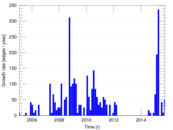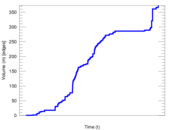### Temporal hop distribution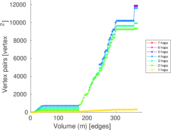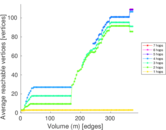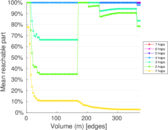### Diameter/density evolution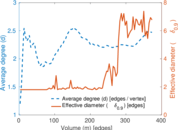### Matrix decompositions plots